International
Tables for
Crystallography
Volume B
Reciprocal space
Edited by U. Shmueli

International Tables for Crystallography (2006). Vol. B, ch. 1.3, pp. 27-28   | 1 | 2 |

## Section 1.3.2.2.5. Tensor products. Fubini's theorem

G. Bricognea

aMRC Laboratory of Molecular Biology, Hills Road, Cambridge CB2 2QH, England, and LURE, Bâtiment 209D, Université Paris-Sud, 91405 Orsay, France

#### 1.3.2.2.5. Tensor products. Fubini's theorem

| top | pdf |

Let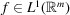,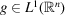. Then the function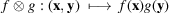is called the tensor product of f and g, and belongs to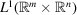. The finite linear combinations of functions of the form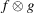span a subspace ofcalled the tensor product of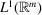and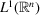and denoted.

The integration of a general function over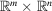may be accomplished in two steps according to Fubini's theorem. Given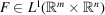, the functions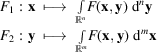exist for almost all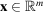and almost all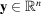, respectively, are integrable, and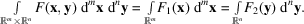Conversely, if any one of the integrals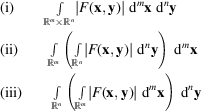is finite, then so are the other two, and the identity above holds. It is then (and only then) permissible to change the order of integrations.

Fubini's theorem is of fundamental importance in the study of tensor products and convolutions of distributions.